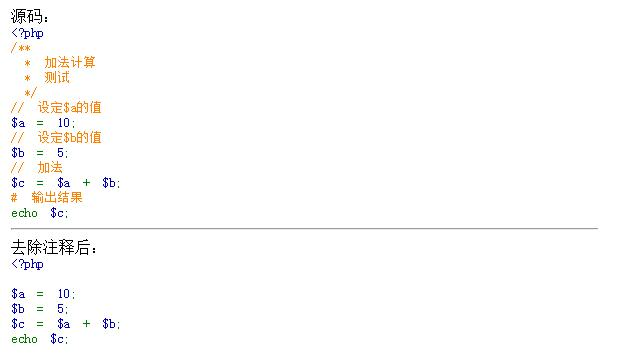# 如何通过php正则去掉注释_编程技术_亿码酷站`<?php/** * 加法计算 * 测试 */// 设定\$a的值\$a = 10;// 设定\$b的值\$b = 5;// 加法\$c = \$a + \$b;# 输出结果echo \$c;`

`echo "源码：<br />";show_source('./a.php');echo "<hr />去除注释后：<br />";highlight_string(removeComment(file_get_contents('./a.php')));/** * 去除PHP代码注释 * @param string \$content 代码内容 * @return string 去除注释之后的内容 */function removeComment(\$content){ return preg_replace("/(\/\*.*\*\/)|(#.*?\n)|(\/\/.*?\n)/s", '', str_replace(array("\r\n", "\r"), "\n", \$content));}``(\/\*.*\*\/) 匹配 /* */(#.*?\n)  匹配 #  遇到第一个回车后结束(\/\/.*?\n) 匹配 //  遇到第一个回车后结束`

`<?php  \$s = '/*aaaa sss */*/';  preg_match('@/\*.*?\*/@s', \$s, \$match);  var_dump(\$match);`

`array(1) { => string(15) "/*aaaa sss */"}`

`<?php \$str = "  a = b; //asdf //saf b = c; //asdf   "; \$reg = '@\s*//.*\$@m';  \$res = preg_replace(\$reg,'',\$str);  echo \$res;`

`a = b; b = c;`

`local test = "int x; /* xx */ xx*/ int y; /* y */"  function string.gfind(test, patten)  local i,j = 0,0;  return function()   i,j = string.find(test, patten, j+1)   if(i == nil) then -- end find    return nil    end    return string.sub(test, i, j)  end  end   for v in string.gfind(test, "/%*.-%*/") do  print(v) end`

/* xx */
/* y */

phpstorm 正则匹配删除注释行（替换注释行为空行）

1. ctrl + F , 输入正则表达式`：(\/\/.*\$)|(\/\*(.|\s)*?\*\/)`

2. ctrl + R, 不输入：

3. 点击后面的 Replace all

1.ctrl + F, 输入正则表达式： `^\n`

2.ctrl + R, 不输入

3.点击后面的 Replace all

1.ctrl + F, 输入正则表达式：` \s\n`

2.ctrl + R, 不输入

3.点击后面的 Replace all

php怎么将图片转二进制字符串？

## java如何进行正则表达式匹配_亿码酷站_编程开发技术教程

java如何进行正则表达式匹配_亿码酷站_编程开发技术教程